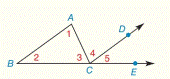Chapter 2.CR, Problem 17CR### Elementary Geometry for College St...

6th Edition
Daniel C. Alexander + 1 other
ISBN: 9781285195698

#### Solutions

Chapter
Section### Elementary Geometry for College St...

6th Edition
Daniel C. Alexander + 1 other
ISBN: 9781285195698
Textbook Problem
1 views

# Given: A B ¯ ∥ C D → m ∠ 2 = x 2 − 3 x + 4 m ∠ 1 = 17 x − x 2 − 5 m ∠ A C E = 111 ° Find: m ∠ 3 , m ∠ 4 , and m ∠ 5To determine

To find:

The value of x.

Explanation

Given:

The given statement is,

AB¯CDm2=x23x+4m1=17xx25mACE=111°

Figure (1)

Concept:

The measure of an exterior angle of a triangle equals the sum of the measures of the two nonadjacent interior angles.

Calculation:

The given statement is,

AB¯CDm2=x23x+4m1=17xx25mACE=111°

ACE is an exterior angle of ΔABC

### Still sussing out bartleby?

Check out a sample textbook solution.

See a sample solution

#### The Solution to Your Study Problems

Bartleby provides explanations to thousands of textbook problems written by our experts, many with advanced degrees!

Get Started

#### Convert the expressions in Exercises 6584 to power form. 35x2

Finite Mathematics and Applied Calculus (MindTap Course List)

#### In Exercise 11-14, factor the expression. x2+5x+6

Calculus: An Applied Approach (MindTap Course List)

#### Which curve is simple but not closed?

Study Guide for Stewart's Multivariable Calculus, 8th

#### True or False: If f′(c) = 0 and f″(c) < 0, then c is a local minimum.

Study Guide for Stewart's Single Variable Calculus: Early Transcendentals, 8th